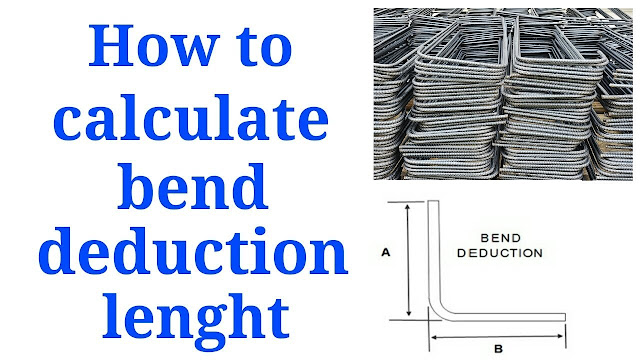How to calculate the bend deduction length of bars

# How to calculate the bend deduction length of barsHow to calculate the bend deduction length of bars

The bends deduction length was to be determined according to their angles. in this lecture the bend reduction length was to be determined. We use different angles for different purposes..
Related Topics:

Continue Reading about the "How to calculate the bend deduction length of bars" on the next page below

1.